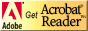645:571 Computational Mathematics I - Fall 2020
Department of Mathematical Sciences
Rutgers University, Camden, NJ

• Textbooks (optional):
• E. Suli and D. Mayers, An Introduction to Numerical Analysis, Cambridge University Press, 2003. ISBN-10: 052100794, ISBN-13: 978-0521007948
• A. Ralston and P. Rabinowitz, A First Course in Numerical Analysis, Second Edition, Dover Publications. ISBN-10: 048641454X, ISBN-13: 978-0486414546
• Class meetings:  Wednesdays 6:00PM - 8:50PM  Online
• Instructor: Dr. Steve Alessandrini, sma@rutgers.edu
• Office hours: Before class.
• Prerequisites:
• Calculus.
• Knowledge of C++.  Tutorial,  SimpleExample.cpp
• C++ Style Guide for Scientific Computing
• It is preferred that this first semester course 645:571 is taken before the second semester course 645:572.
• Course description:
This one-year sequence covers various numerical techniques for solving mathematical problems on a computer.  The first semester 645:571 covers the IEEE internal representation of floating point numbers, interpolation, root finding, numerical integration, numerical differentiation, and function minimization.  The second semester 645:572 covers numerical linear algebra and the numerical solution of differential equations.  The material is presented so that topics build on one another and applications are given to illustrate the use of the techniques.
• Notes and Examples: (PDF Format)• Chapter 1:  Introduction
• Part 1: pp 1 - 13
• Example demonstrating why not to use pow for square roots and squaring: PowTest.cpp
• Chapter 2:  Interpolation
• Part 2: pp 14 - 24
• Part 3: pp 25 - 36
• MATLAB Examples
• RungeExample.m
• PWLinear.m
• Hermite.m
• Spline.m
• RungeExampleSplineEven.m
• RungeExampleSplineOdd.m
• KeyframeAnimation.m
• Cubic Spline Application: How fast could Usain Bolt have run?
• H. K. Eriksen, J. R. Kristiansen, O. Langangen, and I. K. Wehus, "How fast could Usain Bolt have run? A dynamical study," American Journal of Physics, 77, 224-228 (2009).
• SmoothSpline.m
• CubicSplineExample.m
• LeastSquaresExample.m
• polyfitweighted.m
• Smoothing Spline References:
• C. H. Reinsch, "Smoothing by Spline Functions," Numerische Mathematik, 10 (1967), 177-183.
• C. H. Reinsch, "Smoothing by Spline Functions. II," Numerische Mathematik, 16 (1971), 451-454.
• Chapter 3:  Roots of Equations
• Part 4: pp 37 - 45
• Part 5: pp 46 - 60
• MATLAB Examples
• NewtonSqrtExample.m
• CircleFit.m
• Chapter 4:  Numerical Integration
• Part 6: pp 61-70
• Part 7: pp 71-85
• Part 8: pp 86-92
• MATLAB Examples
• Midpoint.m
• Trapezoidal.m
• Simpson.m
• IntegralTest.m
• H. Yserentant, "A remark on the numerical computation of improper integrals," Computing, Vol. 30, N0. 2, (1983), pp. 179-183.
• Chapter 5:  Numerical Differentiation
• Part 9: pp 93-99
• W. Squire and G. Trapp, "Using Complex Variables to Estimate Derivatives of Real Functions," SIAM Review, Vol. 40, No. 1, (March 1998), pp. 110-112.
• K.-L. Lai, J. L. Crassidis, Y. Cheng, and J. Kim, "New Complex-Step Derivative Approximations with Applications to Second-Order Kalman Filtering," 2005 AIAA Guidance,Navigation, and Control Conference and Exhibit, San Francisco, CA, USA, 15-18 August 2005, pp, 1-17.
• MATLAB Example
• TestComplexStepDerivative.m
• Bibliography
• Homework Assignments: See Canvas
• Notes:
• First Class: Wednesday 09/02/2020
• No Class: Wednesday 11/25/2020 - Thanksgiving Break
• Last Regular Class: Wednesday 12/9/2020
• Tentative Final Exam Period: Wednesday 12/16/2020 6pm-9pm

• Rutgers University Academic Integrity Policy

Last Revised: 11:19AM   8/01/2020